Test: Stability Analysis of Linear Control Systems - 1

# Test: Stability Analysis of Linear Control Systems - 1 - Electrical Engineering (EE)

Test Description

## 10 Questions MCQ Test GATE Electrical Engineering (EE) 2024 Mock Test Series - Test: Stability Analysis of Linear Control Systems - 1

Test: Stability Analysis of Linear Control Systems - 1 for Electrical Engineering (EE) 2023 is part of GATE Electrical Engineering (EE) 2024 Mock Test Series preparation. The Test: Stability Analysis of Linear Control Systems - 1 questions and answers have been prepared according to the Electrical Engineering (EE) exam syllabus.The Test: Stability Analysis of Linear Control Systems - 1 MCQs are made for Electrical Engineering (EE) 2023 Exam. Find important definitions, questions, notes, meanings, examples, exercises, MCQs and online tests for Test: Stability Analysis of Linear Control Systems - 1 below.
Solutions of Test: Stability Analysis of Linear Control Systems - 1 questions in English are available as part of our GATE Electrical Engineering (EE) 2024 Mock Test Series for Electrical Engineering (EE) & Test: Stability Analysis of Linear Control Systems - 1 solutions in Hindi for GATE Electrical Engineering (EE) 2024 Mock Test Series course. Download more important topics, notes, lectures and mock test series for Electrical Engineering (EE) Exam by signing up for free. Attempt Test: Stability Analysis of Linear Control Systems - 1 | 10 questions in 30 minutes | Mock test for Electrical Engineering (EE) preparation | Free important questions MCQ to study GATE Electrical Engineering (EE) 2024 Mock Test Series for Electrical Engineering (EE) Exam | Download free PDF with solutions
 1 Crore+ students have signed up on EduRev. Have you?
Test: Stability Analysis of Linear Control Systems - 1 - Question 1

### The presence of non-linearities in a control system tends to introduce

Test: Stability Analysis of Linear Control Systems - 1 - Question 2

### Which one of the following is not a property of the Liapunov function?

Test: Stability Analysis of Linear Control Systems - 1 - Question 3

### The number of sign changes in the Routh’s array indicates the number of roots lying in the

Detailed Solution for Test: Stability Analysis of Linear Control Systems - 1 - Question 3

Number of sign changes in the first column of Routh’s array indicates the number of closed loop poles or roots of characteristic equation lying in the right half of s-plane.

Test: Stability Analysis of Linear Control Systems - 1 - Question 4

Assertion (A): A system is said to be stable if the impulse response approaches zero for sufficiently large time.
Reason (R): If the impulse response approaches infinity for sufficiently large time, the system is said to be unstable

Detailed Solution for Test: Stability Analysis of Linear Control Systems - 1 - Question 4

Both assertion and reason are individually true. The correct reason for assertion is BIBO stability criteria,

Test: Stability Analysis of Linear Control Systems - 1 - Question 5

The open loop transfer function of a unity feedback system is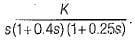What is the range of value of K so that the closed loop system is absolutely stable?

Detailed Solution for Test: Stability Analysis of Linear Control Systems - 1 - Question 5

The characteristic equation is,
1 + G(s)H(s) = 0For absolute stability, we have: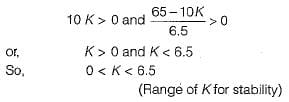Test: Stability Analysis of Linear Control Systems - 1 - Question 6

Assertion (A): If the system is stable we can determine the relative stability by the settling time of the system.
Reason (R): If the settling time is less than that of the other system then the system is said to be relatively more stable.

Detailed Solution for Test: Stability Analysis of Linear Control Systems - 1 - Question 6

The settling time is inversely proportional to the real part of the dominant roots. If the settling time is less compared to other system, then the system will be relatively more stable

Test: Stability Analysis of Linear Control Systems - 1 - Question 7

If the unit step response of a system is given by c(t) = -1.5 + et + 0.5e-2f, then the system will be

Detailed Solution for Test: Stability Analysis of Linear Control Systems - 1 - Question 7

Given, step response: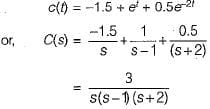∴  Transfer function is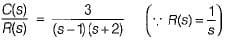Since one of the closed loop pole (s = 1) lies in RH s-plane, therefore given system is unstable.

Test: Stability Analysis of Linear Control Systems - 1 - Question 8

The characteristic equation of a servo system is given by
a0s4 + a1s3 + a2s2 + a3s + a4 = 0
Now, consider the following conditions required to be satisfied by the coefficient of the above characteristic equation for the system to be stable:
1. a1 > 0 and a4 > 0
2. a1 < 0 and a4 > 0
3. a1a2< a0a3
4. a1a2 > a0a
5.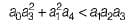6.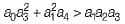Which of the above conditions holds true for the given system to be stable?

Detailed Solution for Test: Stability Analysis of Linear Control Systems - 1 - Question 8

The Rouths array is formed as follows: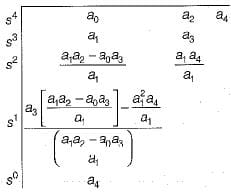For the system to be stable, there should not be any sign change in the first column of Routh’s array.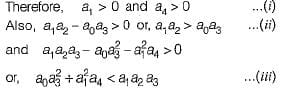Hence, above conditions must hold for the given system to be stable.

Test: Stability Analysis of Linear Control Systems - 1 - Question 9

The open loop transfer function of a unity feedback control system is given by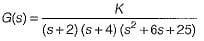​The oscillating frequency of the given system is

Detailed Solution for Test: Stability Analysis of Linear Control Systems - 1 - Question 9

The characteristic equation of given system is: 1 + G(s) = 0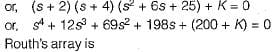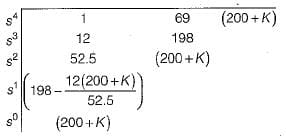Given system will be stable when,
200 + K > 0 or K > -200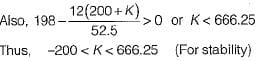System will oscillate when K = Kmar = 666.25
Auxiliary equation is : 52.5 s2 + (200 + K) = 0
or, 52.5 s2 = - 866.25 or, s2 = -16.5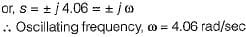Test: Stability Analysis of Linear Control Systems - 1 - Question 10

The characteristic equation of a system is given by s3 + s + 2 - 0.

What are the number of roots in the right half s-plane and on the jω-axis respectively?

Detailed Solution for Test: Stability Analysis of Linear Control Systems - 1 - Question 10

Routh’s array is formed as shown below,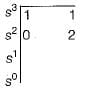Replacing ε in place of 0 in 1st column, we have: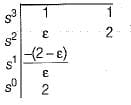To check for sign change: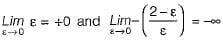Thus, there are two sign changes in 1st column of Routh’s array (+0 to -∞ and -∞ to + 2).
Hence, number of roots in RH s-plane = 2.
Number of roots in LH s-plane = 1.
Number of roots on the jω-axis = 0.

## GATE Electrical Engineering (EE) 2024 Mock Test Series

23 docs|285 tests
Information about Test: Stability Analysis of Linear Control Systems - 1 Page
In this test you can find the Exam questions for Test: Stability Analysis of Linear Control Systems - 1 solved & explained in the simplest way possible. Besides giving Questions and answers for Test: Stability Analysis of Linear Control Systems - 1, EduRev gives you an ample number of Online tests for practice

## GATE Electrical Engineering (EE) 2024 Mock Test Series

23 docs|285 tests